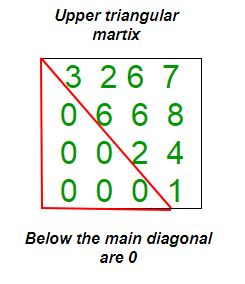GeeksforGeeks App
Open AppBrowser
Continue

# Php Program to check if matrix is upper triangular

Given a square matrix and the task is to check the matrix is in upper triangular form or not. A square matrix is called upper triangular if all the entries below the main diagonal are zero.Examples:

```Input : mat = {{1, 3, 5, 3},
{0, 4, 6, 2},
{0, 0, 2, 5},
{0, 0, 0, 6}};
Output : Matrix is in Upper Triangular form.

Input : mat = {{5, 6, 3, 6},
{0, 4, 6, 6},
{1, 0, 8, 5},
{0, 1, 0, 6}};
Output : Matrix is not in Upper Triangular form.```

## PHP

 ``

Output:

`Yes`

Time Complexity: O(n2), where n represents the number of rows and columns of the matrix.
Auxiliary Space: O(1), no extra space is required, so it is a constant.

Please refer complete article on Program to check if matrix is upper triangular for more details!

My Personal Notes arrow_drop_up